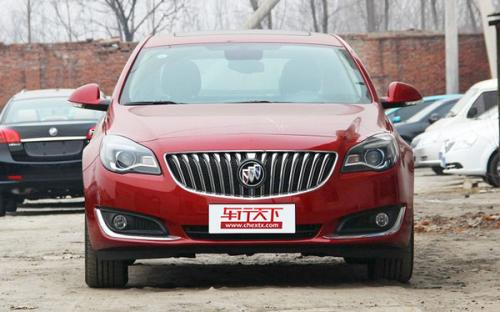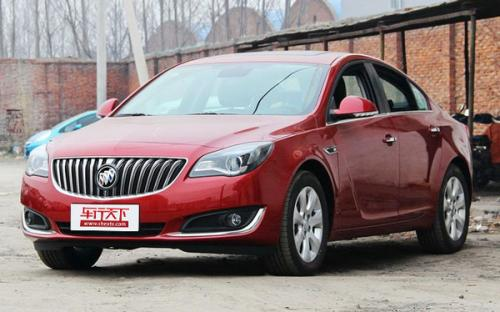### 通用别克 君威5 种颜色可选2015款最低售价：17.89 万元起

4843(mm)1856(mm)1484(mm)##### 配置亮点：
• 胎压监测装置

• ISOFIX儿童座椅接口

• 车身稳定控制(ESC/ESP/DSC等)

• 电动天窗

• 定速巡航

• 后倒车雷达

• 真皮座椅

• GPS导航系统

• 氙气大灯

• 后视镜加热

• 提交
2015款 1.6T 精英技术型 (111张)

通用别克 君威 绕车实拍• 通用别克 君威 在售车型

排量 车型 厂商指导价 本地最低报价 购车工具
2.0L
2.0L 领先时尚型 6挡手自一体
17.89万
17.89万

2.0L 精英时尚型 6挡手自一体
19.39万
19.39万

2.4L
2.0L 领先时尚型 6挡手自一体
17.89万
17.89万

2.0L 精英时尚型 6挡手自一体
19.39万
19.39万

1.6T
1.6T 领先技术型 6挡手自一体
18.99万
18.99万

1.6T 时尚技术型 6挡手自一体
19.99万
19.99万

1.6T 精英技术型 6挡手自一体
20.99万
20.99万

2.0T GS豪情运动版 6挡手自一体
23.99万
23.99万

2.0T GS燃情运动版 6挡手自一体
25.99万
25.99万

2.0T GS纵情运动版 6挡手自一体
29.99万
29.99万

2.0T
1.6T 领先技术型 6挡手自一体
18.99万
18.99万

1.6T 时尚技术型 6挡手自一体
19.99万
19.99万

1.6T 精英技术型 6挡手自一体
20.99万
20.99万

2.0T GS豪情运动版 6挡手自一体
23.99万
23.99万

2.0T GS燃情运动版 6挡手自一体
25.99万
25.99万

2.0T GS纵情运动版 6挡手自一体
29.99万
29.99万

通用别克 君威 经销商

查看更多 >>

### 通用别克 君威 动力加速

君威 0-100公里加速时间分布在 7.3-11.7秒 属于 运动级

动力级别 加速时间 车型
民用级(3款)
11.7s
2.0L 领先时尚型2.0L 精英时尚型
9.5s
2.4L SIDI精英时尚型2.4L SIDI豪华时尚型
9.8s
1.6T 领先技术型1.6T 时尚技术型1.6T 精英技术型
运动级(1款)
7.3s
2.0T GS豪情运动版2.0T GS燃情运动版2.0T GS纵情运动版

通用别克 君威 视频

通用别克 君威 新闻资讯

# 君威1.6T精英运动版上市 仅售20.99万

车系文章 超过2520次关注

上海通用汽车正式推出2014款别克君威1.6T精英运动版，售价20.99万元。这款车型搭载1.6T涡轮增压发动机和6速手自一体变速箱。

# 别克新款君威/君威GS 演绎全新时尚

本地资讯 超过3039次关注

新款君威对别克新一代设计语言进行了全新的时尚演绎，采用加大的新型直瀑式格栅、一体式前后保险杠、展翼式日间行车灯、贯通式车尾镀铬饰条以及全新的座舱设计等等。

# 君威有少量现车 购车最高优惠2.3万元

车系文章 超过2431次关注

近日，编辑从深圳市大兴汽车有限公司了解到，2014款君威在店内有少量现车，目前购车现金最高优惠2.3万元。

# 专治各种不服10 GS挑战CC北京金港圈速

车系文章 超过2742次关注

GS和CC一直是两个针锋相对的对手，都有流线/动感的外形，并且动力及底盘配置都不差，只不过之前的日子里，没机会、没场合外加没人挑明不服气，于是，到底“全面运动...

# 解读低配车 实拍君威2.0L 领先时尚型

车系文章 超过3632次关注

当这一代别克君威发布时，它彻底颠覆了美国车在我们心中的印象，少了几分粗犷，多了一些细腻，流畅动感的造型很有欧洲车的感觉，这也让它迅速得到了消费者的认可。...

# 操控满意保养贵 别克君威车主调查

车系文章 超过2437次关注

从一家申请破产保护的企业到目前的全球销量第一，通用近五年的市场表现可谓是翻天覆地。是什么让这位汽车巨人重振雄风呢？每个人可能会有自己的答案，什么经营管理...

# T军团集结号君威GS试驾会

本地资讯 超过2646次关注

2014年3月30日上海通用别克在中原福塔举行“T”军团集结号别克君威对比试驾活动，届时这款让世人“一再心动”的别克君威和君威GS将在别克粉丝们面前展非凡的气度与...

# 别克君威最高直降2万元

本地资讯 超过2581次关注

旭龙别克车展价提前享，别克君威最高直降2万元，电话预约到店购车还有惊喜等你来拿，3月最后一周，6万定额信贷享0息还有一周时间，没有房产一样可以分期购车，0担保...

猜你喜欢

﻿
• 快速找车
• 选择品牌
• 选择品牌
• A  奥迪
• A  阿斯顿·马丁
• A  阿尔法·罗密欧
• B  宝沃
• B  布加迪
• B  巴博斯
• B  保时捷
• B  宾利
• B  奔驰
• B  宝马
• B  本田
• B  别克
• B  标致
• B  比亚迪
• B  宝骏
• B  北汽制造
• B  北汽新能源
• B  北汽幻速
• B  北汽威旺
• B  北京汽车
• B  奔腾
• B  北汽绅宝
• C  长安
• C  长安商用
• C  长城
• C  昌河
• D  大众
• D  道奇
• D  DS
• D  东南
• D  东风风神
• D  东风风行
• D  东风小康
• D  东风风度
• D  东风
• F  福特
• F  丰田
• F  菲亚特
• F  法拉利
• F  福田
• F  福迪
• F  福汽启腾
• G  观致
• G  广汽传祺
• G  广汽吉奥
• G  GMC
• H  红旗
• H  汉腾汽车
• H  哈弗
• H  哈飞
• H  海格
• H  海马
• H  华颂
• H  黄海
• H  华泰
• H  恒天
• J  吉利汽车
• J  捷豹
• J  Jeep
• J  江淮
• J  江铃
• J  金杯
• J  九龙
• J  金旅
• K  凯翼
• K  凯迪拉克
• K  克莱斯勒
• K  科尼塞克
• K  卡威
• K  开瑞
• L  路虎
• L  林肯
• L  劳斯莱斯
• L  兰博基尼
• L  雷克萨斯
• L  铃木
• L  雷诺
• L  理念
• L  力帆
• L  莲花汽车
• L  猎豹
• L  路特斯
• L  陆风
• M  马自达
• M  MG
• M  MINI
• M  玛莎拉蒂
• M  摩根
• M  迈凯轮
• N  纳智捷
• O  欧宝
• O  讴歌
• O  欧朗
• Q  奇瑞
• Q  起亚
• Q  启辰
• R  日产
• R  荣威
• R  瑞麒
• S  三菱
• S  斯威汽车
• S  萨博
• S  smart
• S  斯柯达
• S  斯巴鲁
• S  思铭
• S  双龙
• S  上汽大通
• S  双环
• T  特斯拉
• T  腾势
• W  沃尔沃
• W  五菱汽车
• W  五十铃
• W  威兹曼
• W  威麟
• X  现代
• X  雪佛兰
• X  雪铁龙
• X  西雅特
• Y  一汽
• Y  英菲尼迪
• Y  英致
• Y  依维柯
• Y  野马汽车
• Y  永源
• Z  众泰
• Z  中华
• Z  中兴
• Z  知豆
• 选择车系
• 选择车系
• 车型对比
• 选择品牌
• 选择品牌
• A  奥迪
• A  阿斯顿·马丁
• A  阿尔法·罗密欧
• B  宝沃
• B  布加迪
• B  巴博斯
• B  保时捷
• B  宾利
• B  奔驰
• B  宝马
• B  本田
• B  别克
• B  标致
• B  比亚迪
• B  宝骏
• B  北汽制造
• B  北汽新能源
• B  北汽幻速
• B  北汽威旺
• B  北京汽车
• B  奔腾
• B  北汽绅宝
• C  长安
• C  长安商用
• C  长城
• C  昌河
• D  大众
• D  道奇
• D  DS
• D  东南
• D  东风风神
• D  东风风行
• D  东风小康
• D  东风风度
• D  东风
• F  福特
• F  丰田
• F  菲亚特
• F  法拉利
• F  福田
• F  福迪
• F  福汽启腾
• G  观致
• G  广汽传祺
• G  广汽吉奥
• G  GMC
• H  红旗
• H  汉腾汽车
• H  哈弗
• H  哈飞
• H  海格
• H  海马
• H  华颂
• H  黄海
• H  华泰
• H  恒天
• J  吉利汽车
• J  捷豹
• J  Jeep
• J  江淮
• J  江铃
• J  金杯
• J  九龙
• J  金旅
• K  凯翼
• K  凯迪拉克
• K  克莱斯勒
• K  科尼塞克
• K  卡威
• K  开瑞
• L  路虎
• L  林肯
• L  劳斯莱斯
• L  兰博基尼
• L  雷克萨斯
• L  铃木
• L  雷诺
• L  理念
• L  力帆
• L  莲花汽车
• L  猎豹
• L  路特斯
• L  陆风
• M  马自达
• M  MG
• M  MINI
• M  玛莎拉蒂
• M  摩根
• M  迈凯轮
• N  纳智捷
• O  欧宝
• O  讴歌
• O  欧朗
• Q  奇瑞
• Q  起亚
• Q  启辰
• R  日产
• R  荣威
• R  瑞麒
• S  三菱
• S  斯威汽车
• S  萨博
• S  smart
• S  斯柯达
• S  斯巴鲁
• S  思铭
• S  双龙
• S  上汽大通
• S  双环
• T  特斯拉
• T  腾势
• W  沃尔沃
• W  五菱汽车
• W  五十铃
• W  威兹曼
• W  威麟
• X  现代
• X  雪佛兰
• X  雪铁龙
• X  西雅特
• Y  一汽
• Y  英菲尼迪
• Y  英致
• Y  依维柯
• Y  野马汽车
• Y  永源
• Z  众泰
• Z  中华
• Z  中兴
• Z  知豆
• 选择车系
• 选择车系
• 选择车型
• 选择车型
• 意见反馈